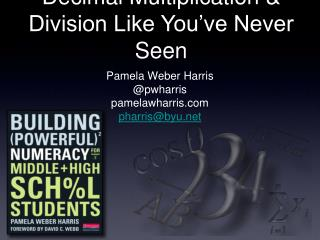# Decimal Multiplication & Division Like You’ve Never Seen - PowerPoint PPT PresentationDownload PresentationDecimal Multiplication & Division Like You’ve Never Seen

Decimal Multiplication & Division Like You’ve Never SeenDownload Presentation## Decimal Multiplication & Division Like You’ve Never Seen

- - - - - - - - - - - - - - - - - - - - - - - - - - - E N D - - - - - - - - - - - - - - - - - - - - - - - - - - -
##### Presentation Transcript

1. Decimal Multiplication & Division Like You’ve Never Seen • Pamela Weber Harris • @pwharris • pamelawharris.com • pharris@byu.net

2. Book Signing • Pamela Weber Harris • Heinemann #516

3. Math is figure-out-able!

4. Counting Additive Multiplicative Proportional Reasoning Algebraic Reasoning

5. 11 x 12

6. 132 ... Um, I knew 10 x 12 = 120, and um, then I just add 12 because 1 x 12 = 12 and um I just add 12 to 120 and I got 132.

7. I didn’t ask you if you know it, I asked you what it is.What do you know...and figure it out from there!

8. ONE FACTS ZERO FACTS

9. ONE FACTS ZERO FACTS DOUBLE

10. ONE FACTS ZERO FACTS DOUBLE DOUBLE + ONE

11. ONE FACTS ZERO FACTS DOUBLE DOUBLE + ONE DOUBLE, DOUBLE

12. DOUBLE, DOUBLE DOUBLE ONE FACTS ZERO FACTS DOUBLE DOUBLE + ONE DOUBLE, DOUBLE

13. DOUBLE, DOUBLE DOUBLE ONE FACTS ZERO FACTS DOUBLE DOUBLE + ONE DOUBLE, DOUBLE TENS

14. DOUBLE, DOUBLE DOUBLE ONE FACTS ZERO FACTS DOUBLE DOUBLE + ONE DOUBLE, DOUBLE NINES TENS

15. DOUBLE, DOUBLE DOUBLE ONE FACTS ZERO FACTS DOUBLE DOUBLE + ONE DOUBLE, DOUBLE NINES 5 IS HALF OF 10 TENS

16. DOUBLE, DOUBLE DOUBLE ONE FACTS ZERO FACTS DOUBLE DOUBLE + ONE DOUBLE, DOUBLE NINES 5 AND ONE MORE 5 IS HALF OF 10 TENS

17. Practice with a Game

18. 2 x 274 x 278 x 2710 x 279 x 275 x 27100 x 2799 x 27 324/27 405/27 Problem Strings

19. 2 x 3.24 x 3.28 x 3.210 x 3.29 x 3.25 x 3.2 15 x 3.2100 x 3.299 x 3.2 44.8/3.2 323.2/3.2 313.6/3.2 480/3.2 Problem Strings

20. Problem Strings 3 x 86 x 48 x 64 x 1216 x 325 x 642.5 x 3.21.8 x 3.5

21. Problem Strings

22. Problem Strings 9 x 109 x 49 x 1410 x 117 x 1117 x 1112 x 161.2 x 16

23. Problem Strings 7 x 607 x 5930 x 1229 x 126 x 46 x 406 x 396 x 0.16 x 4.16 x 3.9

24. Problem Strings 8 x 608 x 598 x 0.18 x 0.28 x 6.28 x 5.848.8 / 847.2 / 8

25. Counting Additive Multiplicative Proportional Reasoning Algebraic Reasoning

26. Not just about any way to do a problem......

27. The Landscape of Learning

28. Math is Figure-out-able • Look to most efficient strategies • Teachers build own numeracy • It’s about relationships: • Among numbers to solve problems • Between teachers and students to build young mathematicians

29. Decimal Multiplication & Division Like You’ve Never Seen • Pamela Weber Harris • facebook.com/numeracy • @pwharris • pamelawharris.com • pharris@byu.net Book signing Heinemann #516